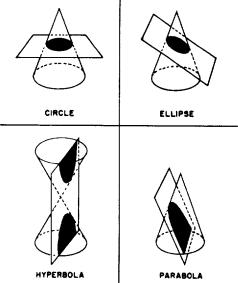Custom SearchCHAPTER 2 CONIC SECTIONS LEARNING OBJECTIVES Upon completion of this chapter, you should be able to do the following: l. Determine the equation of a curve using the locus of the equation. 2. Determine the equation and properties of a circle, a parabola, an ellipse, and a hyperbola. 3. Transform polar coordinates to Cartesian coordinates and viceversa. INTRODUCTION This chapter is a continuation of the study of analytic geometry. The figures presented in this chapter are plane figures, which are included in the general class of conic sections or simply "conics." Conic sections are so named because they are all plane sections of a right circular cone. A circle is formed when a cone is cut perpendicular to its axis. An ellipse is produced when the cone is cut obliquely to the axis and the surface. A hyperbola results when the cone is intersected by a plane parallel to the axis, and a parabola results when the intersecting plane is parallel to an element of the surface. These are illustrated in figure 2-1. When such a curve is plotted on a coordinate system, it may be defined as follows: A conic section is the locus of all points in a plane whose distance from a fixed point is a constant ratio to its distance from a fixed line. The fixed point is the focus, and the fixed line is the directrix.Figure 2-1.-Conic sections. The ratio referred to in the definition is called the eccentricity (e). If the eccentricity is greater than 0 and less than 1, the curve is an ellipse. If e is greater than 1, the curve is a hyperbola. If e is equal to 1, the curve is a parabola. A circle is a special case having an eccentricity equal to 0. It is actually a limiting case of an ellipse in which the eccentricity approaches 0. Thus, ifThe eccentricity, focus, and directrix are used in the algebraic analysis of conic sections and their corresponding equations. The concept of the locus of an equation also enters into analytic geometry; this concept is discussed before the individual conic sections are presented.Integrated Publishing, Inc. - A (SDVOSB) Service Disabled Veteran Owned Small Business